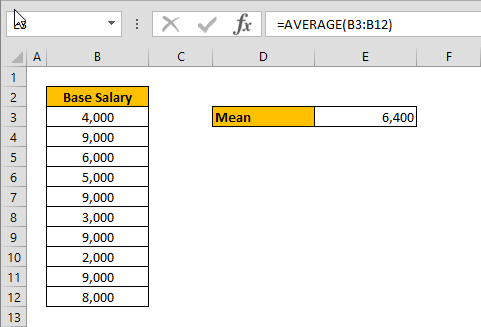Calculating the mean of numbers is one of staples of statistical analysis processes. In this article, we're going to show you how to calculate mean in Excel using the AVERAGE formula.# Syntax

=AVERAGE( array of numbers )

# Steps

1. Begin by creating the formula =AVERAGE(
2. Select your data range that contains the values (i.e. B3:B12)
3. Finish the formula with ) and press the Enter key.

# How to find the mean in Excel

The mean or the statistical mean is essentially means average value and can be calculated by adding data points in a setand then dividing the total, by the number of points. Excel's AVERAGE function does exactly this: sum all the values and divides the total by the count of numbers.

The AVERAGE function can also be configured to ignore empty cells and cells that do not include any numbers. If you want to include empty or invalid values in calculations as zeroes, use the AVERAGEA function. The AVERAGEA function works exactly the same with the AVERAGE.

=AVERAGEA( array of numbers )